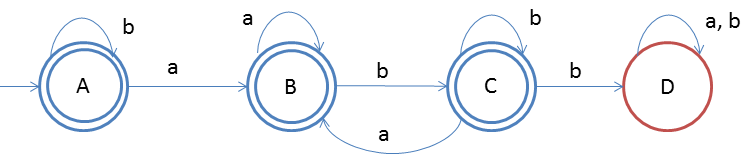# DFA String Examples

We will now discuss about string patterns such as, starting with some symbol, ending with some symbol, etc.

Design a DFA in which every 'a' should never followed by 'bb'
Given: Input alphabet, Σ={a, b}
Language L = {ε, aa, bb, ab, aaa, bbbb, ...}

Clearly the language is infinite because there is infinite number of strings.The idea behind this approach is very simple, follow the steps below and you will understand.

1. Firstly there is ε so make start state as final state
2. And we do not care if string is only 'bbbbb...'(as this doesn't violate given property) so put self-loop on start state itself
3. if a comes then we will move state B and we will make B as final state cause single 'a' also satisfy the property
4. Here is trick part: if b comes after a we should accept it too, so C will be final state too
5. And if 'a' comes at state C then we should direct it to state B as the property of DFA follows
6. But if 'b' comes at state C then it should be rejected, and after that 'a' and 'b' doesn't bother

### Testing

1. Lets take one input string baba
2. Scan string from left to right
3. First input is b, so from state A we will go to state A itself
4. Second input is a, so from state A we will go to state B
5. Third input is b, so from state B we will go to state C
6. Fourth input is a, so from state C we will go to state B(final state)
After end of the string we are at final state so string is accepted.
You may also try some examples of strings to run over this DFA, and you will understand more